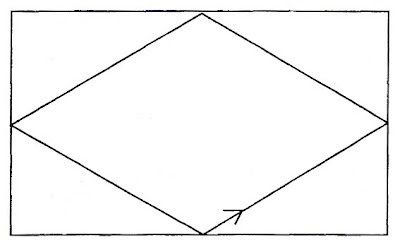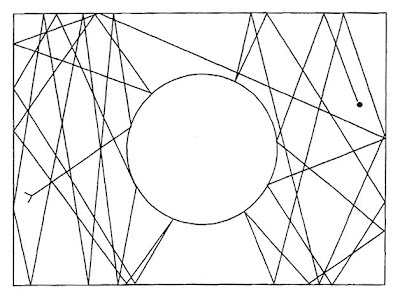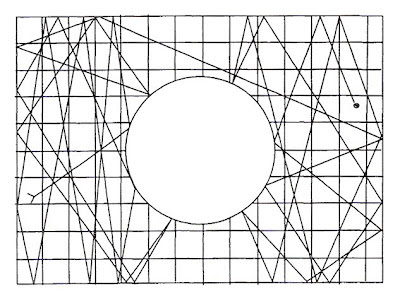## Tuesday, May 20, 2008

### moral certaintySomething is morally certain if its probability comes so close to complete certainty that the difference cannot be perceived. By contrast, something is morally impossible if it has only as much certainty as the amount by which moral certainty falls short of complete certainty. Thus if we take something that possesses 9991000 of certainty to be morally certain, then something that has only 11000 of certainty will be morally impossible.

~ Jacob Bernoulli (1713) Ars Conjectandi

## Sunday, May 18, 2008

### sinai billiards

Billiards is our paradigmatic metaphor for understanding a deterministic universe. This is at least partly due to the fact that motion on a billiards table is quite simple and periodic.Suppose, however, instead of a usual billiards table, we play upon one with a convex obstacle, say a hemisphere, in the middle of the table. Now, the motion of the balls, though still deterministic, exhibits chaotic behavior.The behavior of the balls is still governed by deterministic equations, yet it is no longer simple or periodic.

Consider now the inverse problem. Suppose, rather than knowing these equations, we are observing the table and trying to determine the mathematics which governs the movement of the balls. How might we do this? Since our observations are necessarily of finite accuracy, we might partition the free portion of the table into a finite grid. At various points in time we make observations and record which squares of the grid in which a ball is present. The size of the squares in the grid represents the accuracy of our measurements.In 1976, Y. G. Sinai proved that in this inverse situation, billiard ball behavior governed by a deterministic equation and billiard ball behavior governed by a first order Markov process are indistinguishable. Since a first order Markov process is a probabilistic process which depends only upon the previous square of the grid, it is both indeterministic and, in principle, unpredictable.

Thus, not only is it the case that we are in principle unable to distinguish between a deterministic and an indeterministic universe; but also, even if the universe is deterministic, and we know its initial conditions to an arbitrary degree of accuracy, we will still, in principle, be unable to predict its behavior.

[images from Suppes, Patrick (1999) "The Noninvariance of Deterministic Causal Models"]